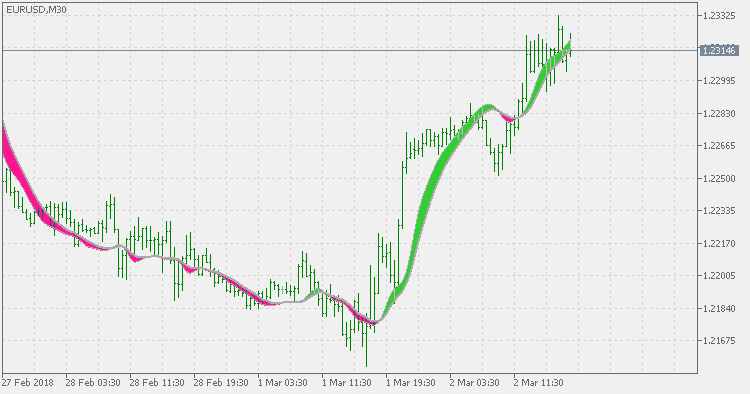Interesting script?
So post a link to it -
let others appraise it

You liked the script? Try it in the MetaTrader 5 terminalInstantaneous Trend Line bands (generalized) - indicator for MetaTrader 5

Views:
2318
Rating:
Published:
2018.03.05 13:40

One of the series of indicators created by John Ehlres. According to Ehlers:

Instantaneous trend Line:

It works because it completely removes the dominant cycle from a smoothing average.

For the mathematically inclined, the Fourier Transform of a rectangular window (formed by a simple average) is a Sin(X)/X distribution. The objective is to place the first null of this distribution exactly at the dominant cycle. For the intuitively inclined, what we are doing is taking a simple average over the full dominant cycle period. In such an average, there are as many sample points above the midpoint as below the midpoint with the result that the sum of all these sample points is zero.

This version extends the Instantaneous Trend Line indicator with a sort of bands to make it easier to spot trend change and to filter out some false signals that can be produced if only the slope of the ITL indicator is takes for a signal. And it also differs from the original in one important part: instead of using fractional alpha parameter for calculation, this version uses period which makes it the same category as averages are and the usage becomes a bit more intuitive than the original version.Instantaneous Trend Line (generalized)

This version differs from the original Instantaneous Trend Line in one important part: instead of using fractional alpha parameter for calculation, this version uses period which makes it the same category as averages are and the usage becomes a bit more intuitive than the original version.Channels

Trading in a channel based on a Moving Average with the period of 220 and three Envelopes with the periods of 220 and different heights. Position trailing.Fractal Adaptive MACD

Fractal Adaptive Moving Average Technical Indicator (FRAMA) was developed by John Ehlers. This indicator is constructed based on the algorithm of the Exponential Moving Average, in which the smoothing factor is calculated based on the current fractal dimension of the price series. The advantage of FRAMA is the possibility to follow strong trend movements and to sufficiently slow down at the moments of price consolidation.ZigZag separate

ZigZag separate is a MetaTrader 5 version of one indicator that was floating around the net as a wonder indicator for MetaTrader 4.